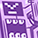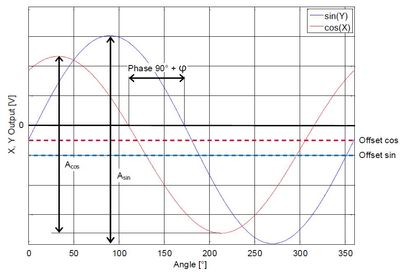3D Hall (Magnetic sensor)Level 1Level 1

# How can I determine an specific angle of a magnet?

in document <Out of Shaft with magnetic 3D sensor>:Out of shaft

"The sensor outputs the magnitude of magnetic field.But the corresponding maximum and zero-crossing points of the SIN and COS signals do not occur at the precise distance of 90°. The difference between X and Y phases is called the orthogonality error.

There is another more accurate way to determine the orthogonality error. The orthogonality can be calculated
out of the magnitude of two 90° angle shifted components. Possible angle combinations are 45° and 135°, 135°
and 225°, 225° and 315° or 315° and 45°."Does "two 90° angle shifted components" mean any angles that has a 90° angle shift?

if not,only the "Possible angle combinations" makes sense,45° and 135° for example,how can I determine the 45° and 135° of a magnet?

1 SolutionModeratorModerator

# Re: How can I determine an specific angle of a magnet?

Hi @Vinolzy,

Thanks for the post and apologies for the delayed response.

Does "two 90° angle shifted components" mean any angles that have a 90° angle shift?

There are three axis X, Y, and Z. When the sensor is X-Y (centered) configuration X-Y will have a 90° phase shift while Bz is zero. This is due to the orientation of the sensor along the X-Y-Z axis.
Please refer to the section 3 Configuration overview in: https://www.infineon.com/dgdl/Infineon-Out_of_Shaft-AN-v01_00-EN.pdf?fileId=5546d46265257de801653898...

if not, only the "Possible angle combinations" makes sense,45°, and 135° for example:

This is to measure the orthogonality error, as the magnitudes of the Bx and By will be the same at 45° and 135° this will help us to calculate the orthogonality error using equations 8 and 9

how can I determine the 45° and 135° of a magnet?
Based on the magnitudes of Bx and By taking one as reference and plot the magnitude measured from the 3D hall sensor. You can use a 3-D simulation tool to simulate the waveform : https://design.infineon.com/3dsim/

Please refer to the section 3 Configuration overview in https://www.infineon.com/dgdl/Infineon-Out_of_Shaft-AN-v01_00-EN.pdf?fileId=5546d46265257de801653898...

Thanks,

KrupashankarModeratorModerator

# Re: How can I determine an specific angle of a magnet?

Hi @Vinolzy,

Thanks for the post and apologies for the delayed response.

Does "two 90° angle shifted components" mean any angles that have a 90° angle shift?

There are three axis X, Y, and Z. When the sensor is X-Y (centered) configuration X-Y will have a 90° phase shift while Bz is zero. This is due to the orientation of the sensor along the X-Y-Z axis.
Please refer to the section 3 Configuration overview in: https://www.infineon.com/dgdl/Infineon-Out_of_Shaft-AN-v01_00-EN.pdf?fileId=5546d46265257de801653898...

if not, only the "Possible angle combinations" makes sense,45°, and 135° for example:

This is to measure the orthogonality error, as the magnitudes of the Bx and By will be the same at 45° and 135° this will help us to calculate the orthogonality error using equations 8 and 9

how can I determine the 45° and 135° of a magnet?
Based on the magnitudes of Bx and By taking one as reference and plot the magnitude measured from the 3D hall sensor. You can use a 3-D simulation tool to simulate the waveform : https://design.infineon.com/3dsim/

Please refer to the section 3 Configuration overview in https://www.infineon.com/dgdl/Infineon-Out_of_Shaft-AN-v01_00-EN.pdf?fileId=5546d46265257de801653898...

Thanks,

Krupashankar